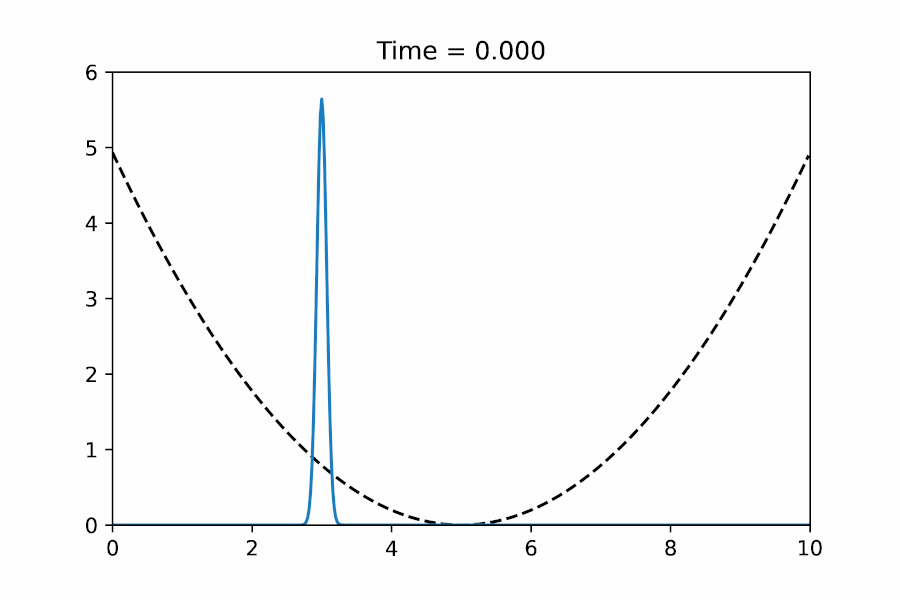# Solving 1-D Schrodinger Equation in PythonSolving 1-D Schrodinger Equation in Python.

As a student majoring in Physics, Quantum Mechanics is an important subject to learn. Solving the time-dependent Schrodinger Equation, thereby seeing the time-evolution of wave-function numerically, can be an important experience to achieve a good understanding of Quantum Dynamics. In this article, I’ll show you how to use python to generate a short animation about a simple-harmonic-oscillator, a wavepacket moving back and forth in a quadratic potential well:A wavepacket moving back and forth in a quadratic potential well

# Discretization (Finite Difference)

This is the one-dimensional time-dependent Schrodinger Equation:

To solve it numerically, we first discretize it in the spatial direction, i.e., using the finite-difference scheme:

The corresponding Laplace operator can be expressed in terms of a matrix D2:

, where the dx is the spacing between discretized spatial grid points.

Since the matrix D2 is a sparse banded matrix, we used the function `scipy.sparse.diags()` to generate the sparse version of it, which gives us better performance.

`dx    = 0.02                       # spatial separationx     = np.arange(0, 10, dx)       # spatial grid points# Laplace Operator (Finite Difference)D2 = scipy.sparse.diags([1, -2, 1],                         [-1, 0, 1],                        shape=(x.size, x.size)) / dx**2`

By using this D2 operator, we may write down the so-called Right-Hand-Side (RHS) of the Schrodinger Equation, `psi_t(t,ψ)`in the discretized form:

`# RHS of Schrodinger Equationhbar = 1def psi_t(t, psi):    return -1j * (- 0.5 * hbar / m * D2.dot(psi) + V / hbar * psi)`

# Time-evolution (Runge–Kutta method)

The function `psi_t(t,ψ)` is the RHS of our system that we will feed into the Initial-Value-Problem solver, `scipy.integrate.solve_ivp()`:

`dt = 0.005  # time interval for snapshotst0 = 0.0    # initial timetf = 1.0    # final timet_eval = np.arange(t0, tf, dt)  # recorded time shots# Solve the Initial Value Problemsol = scipy.integrate.solve_ivp(psi_t,                                 t_span = [t0, tf],                                y0 = psi0,                                 t_eval = t_eval,                                method="RK23")`

# Conservation of Probability

The time evolution driven by the Schrodinger equation guarantees the conservation of probability. This can be verified by printing out the total probability in each snapshot, which is a good sanity check:

`# Print Total Probability (Should = 1)for i, t in enumerate(sol.t):    print(np.sum(np.abs(sol.y[:,i])**2) * dx)`

# Plotting

The simulation result can be plotted by:

`for i, t in enumerate(sol.t):    plt.plot(x, np.abs(sol.y[:,i])**2)`

# Animation

To generate an animation like the one shown at the beginning of this article, we can use the `matplotlib.animation` package:

`import matplotlib.pyplot as pltfrom matplotlib import animationfig = plt.figure()ax1 = plt.subplot(1,1,1)ax1.set_xlim(0, 10)ax1.set_ylim(0, 6)title = ax1.set_title('')line1, = ax1.plot([], [], "k--")line2, = ax1.plot([], [])def init():    line1.set_data(x, V * 0.01)    return line1,def animate(i):    line2.set_data(x, np.abs(sol.y[:,i])**2)    title.set_text('Time = {0:1.3f}'.format(sol.t[i]))    return line1,anim = animation.FuncAnimation(fig, animate, init_func=init,                               frames=len(sol.t), interval=50,                                                blit=True)# Save the animation into a short videoanim.save('sho.mp4', fps=15,           extra_args=['-vcodec', 'libx264'], dpi=600)`

# Complete source (Jupyter notebook)

You may find the complete Jupyter notebook in this Github repository: https://github.com/c0rychu/SchrodingerEq_1D_tutorialhttps://blog.gwlab.page/media/2fea47821f5b57e39dd67a5a5bf8cd99

# Homework?!

Could you try to modify the potential in the code to generate an animation about a wavepacket scattered by a step potential like this?

The solution is here 🙂

# Discussion about the initial condition

Given that the Schrodinger equation is first order in time, we only need to specify ψ(xt=0) as our initial condition. This is very different from the classical wave equation, which is second order in time and we have to specify both ψ(xt=0) and its first time-derivative ∂ψ(xt=0)/∂as our initial condition.

# C++ Version

If you would like to write the whole thing in C++, here is an example code:
https://github.com/c0rychu/SchrodingerEq_1D_tutorial/tree/master/step_potential_cpp
Especially, we wrote the RK4 time-evolution solver part by ourselves while the matrix operations are tackled by the Eigen library.